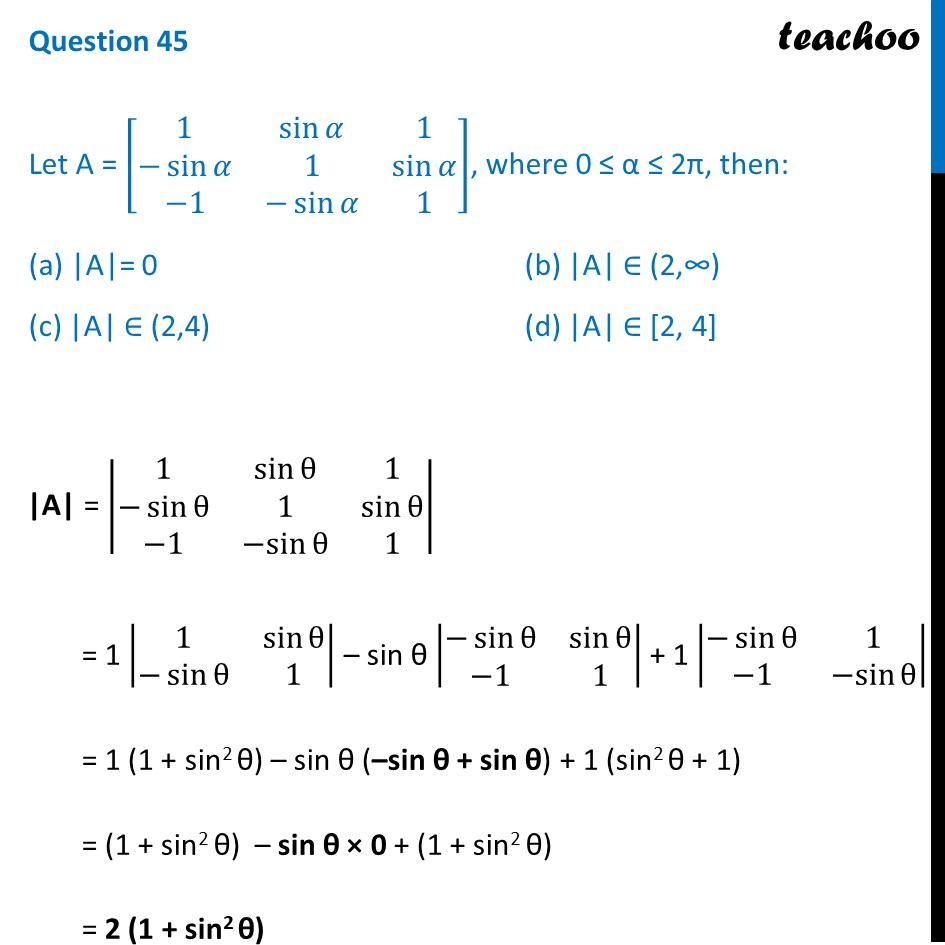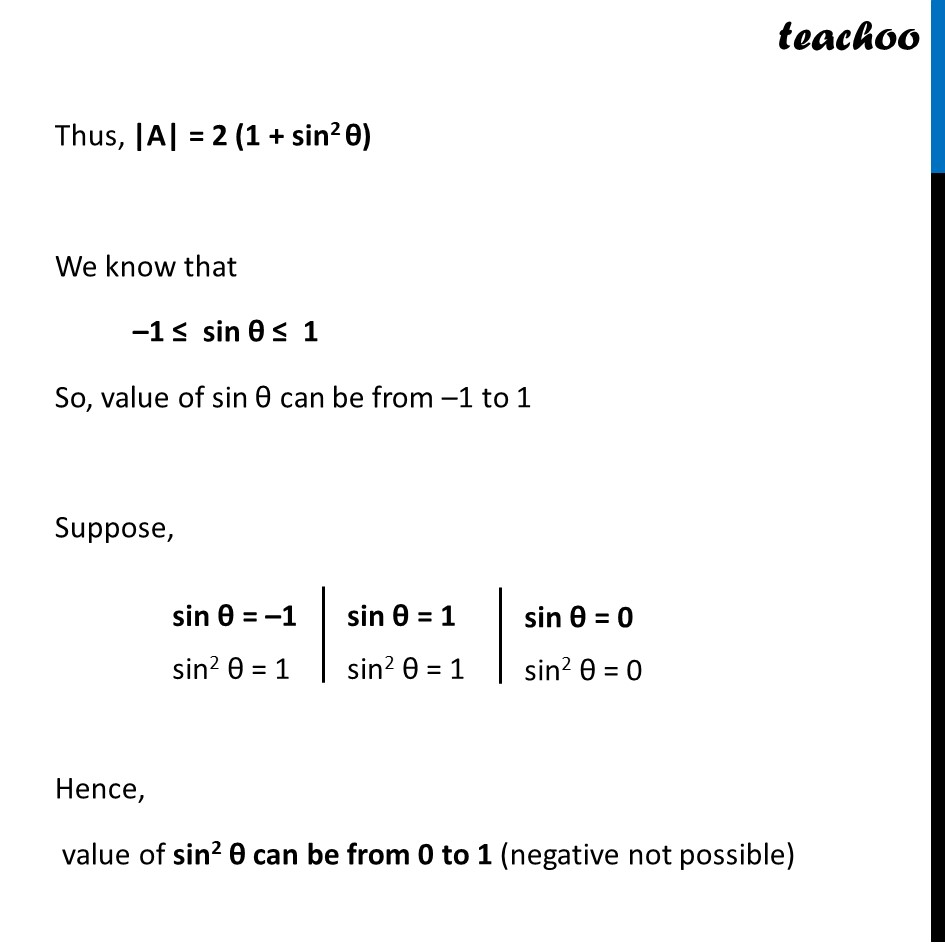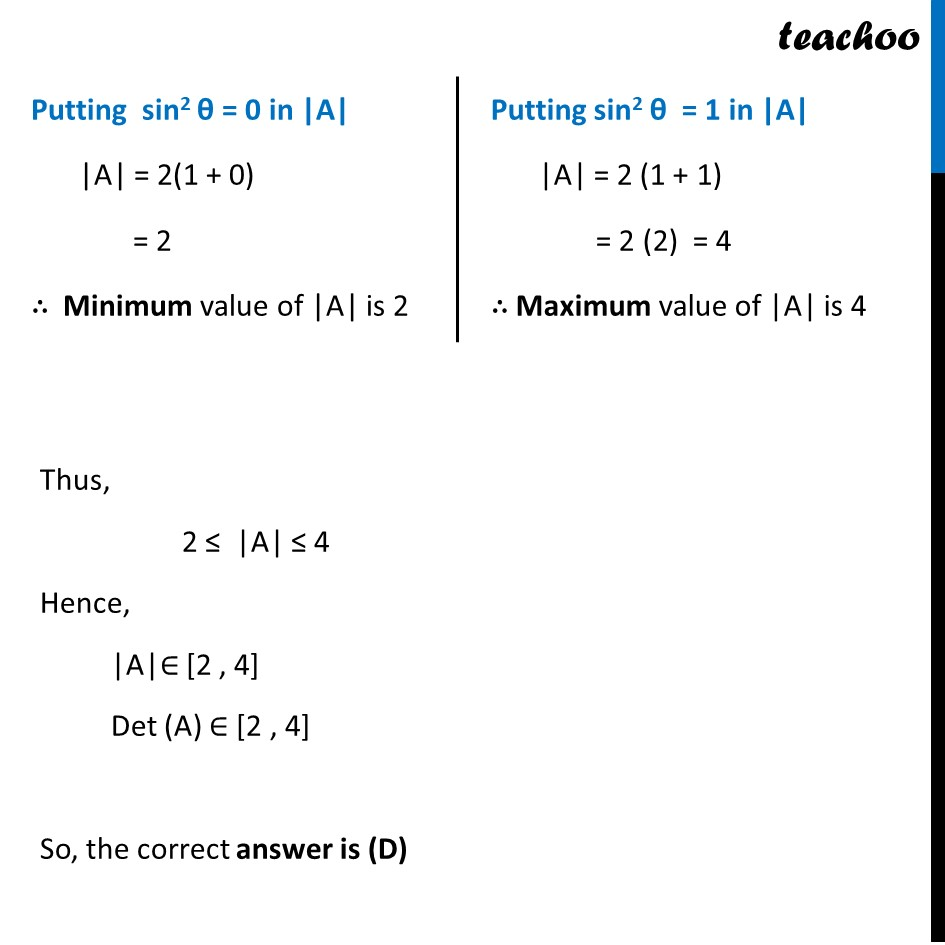CBSE Class 12 Sample Paper for 2022 Boards (MCQ Based - for Term 1)

Class 12
Solutions of Sample Papers and Past Year Papers - for Class 12 Boards

## (c) |A| ∈ (2,4)  (d) |A| ∈ [2, 4]

This question is inspired from Misc 19 (MCQ) - Chapter 4 Class 12 - DeterminantsLearn in your speed, with individual attention - Teachoo Maths 1-on-1 Class

### Transcript

Question 45 Let A = [■8(1&sin⁡𝛼&1@−sin⁡𝛼&1&sin⁡𝛼@−1&−sin⁡𝛼&1)], where 0 ≤ α ≤ 2π, then: (a) |A|= 0 (b) |A| ∈ (2,∞) (c) |A| ∈ (2,4) (d) |A| ∈ [2, 4] |A| = |■8(1&sin⁡θ&1@−sin⁡θ&1&sin⁡θ@−1&〖−sin〗⁡θ&1)| = 1 |■8(1&sin⁡θ@−sin⁡θ&1)| – sin θ |■8(−sin⁡θ&sin⁡θ@−1&1)| + 1 |■8(−sin⁡θ&1@−1&〖−sin〗⁡θ )| = 1 (1 + sin2 θ) – sin θ (–sin θ + sin θ) + 1 (sin2 θ + 1) = (1 + sin2 θ) – sin θ × 0 + (1 + sin2 θ) = 2 (1 + sin2 θ) Thus, |A| = 2 (1 + sin2 θ) We know that –1 ≤ sin θ ≤ 1 So, value of sin θ can be from –1 to 1 Suppose, Hence, value of sin2 θ can be from 0 to 1 (negative not possible) Putting sin2 θ = 0 in |A| |A| = 2(1 + 0) = 2 ∴ Minimum value of |A| is 2 Putting sin2 θ = 1 in |A| |A| = 2 (1 + 1) = 2 (2) = 4 ∴ Maximum value of |A| is 4